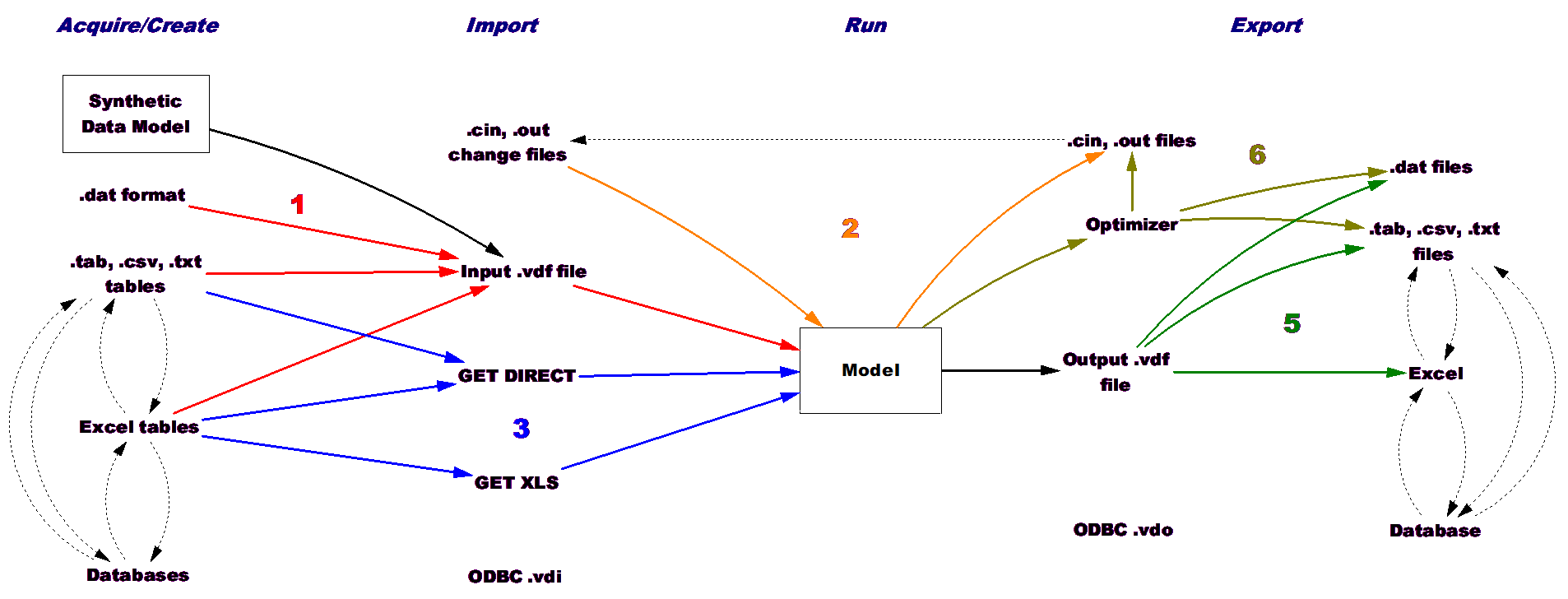# Vensim Help

 Navigation: Reference Guide > Functions > Dynamic Functions SMOOTH(input,delay time) exponential SMOOTH SMOOTHI(input,delay time,initial value) exponential SMOOTH with Initial

Returns a exponential smooth of the input.  Equivalent to the equations:

SMOOTH=INTEG((input-SMOOTH)/delay time,input)

SMOOTHI=INTEG((input-SMOOTHI)/delay time,initial value)

 Units: SMOOTH(units,time) --> units
 SMOOTHI(units,time,units) --> units

The input units match the output units.  The units of delay time must match those of TIME  STEP.  For SMOOTHI units for the initial value must match those of the input.

ExampleS = STEP(10,40)

SS = SMOOTH(S,20)

SSI = SMOOTHI(S,20,5)